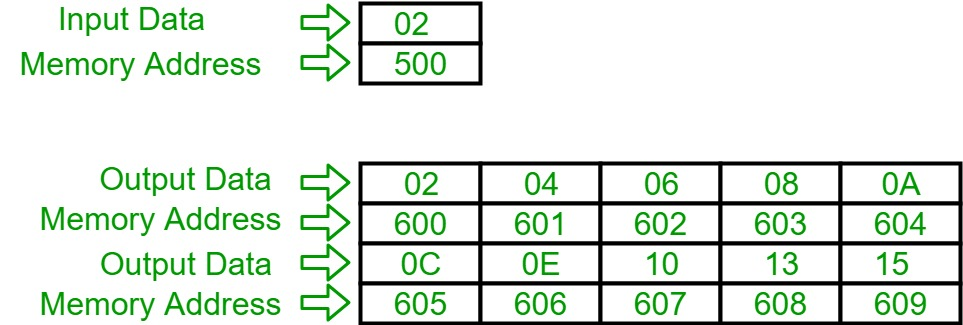# 8086 program to print the table of input integer

Problem – Write an assembly language program in 8086 to print the table of input integer.

Assuption – Suppose the inputted number is at memory location 500 and the table will be printed from starting location 600 till 609 in hexadecimal.

Example –Algorithm –

2. Store 00 in CH register.
3. Increment value of CH by 1 and move the content of [SI] into AH register.
4. Multiply content of AL and CH and store it in AX and then move content of AL into [DI], then increment value of DI by 1.
5. Compare the value of CH and 0A, if not equal then go to step number 3 otherwise halt the program.

Program –

400 MOV SI, 500 SI<-500
403 MOV DI, 600 DI<-600
406 MOV CH, 00 CH<-00
408 INC CH CH<-CH+1
409 MOV AL, [SI] AL<-[SI]
40B MUL CH AX<-AL*CH
40D MOV [DI], AL [DI]<-AL
40F INC DI DI<-DI+1
410 CMP CH, 0A CH-0A
415 HLT Terminates the program

Explanation –

1. MOV SI, 500: load 500 in SI.
2. MOV DI, 600: load 600 in DI.
3. MOV CH, 00: load 00 data in CH register.
4. INC CH: increment the value inside CH register by 1.
5. MOV AL, SI: move the content of SI into AL register.
6. MUL CH: multiply the contents of AL and CH register and store in AX register.
7. MOV [DI], AL: move the contents of AL register into [DI].
8. INC DI: increment the value of DI by 1.
9. CMP CH, 0A: subtract data inside CH register and 0A.